Next: Jurin's Law Up: Surface Tension Previous: Capillary Length

Angle of Contact

Suppose that a liquid/air interface is in contact with a solid, as would be the case for water in a glass tube, or a drop of mercury resting on a table. Figure 3.2 shows a section perpendicular to the edge at which the liquid,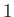, the air,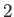, and the solid,, meet. Suppose that the free energies per unit area at the liquid/air, liquid/solid, and air/solid interfaces are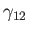,, and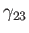, respectively. If the boundary between the three media is slightly modified in the neighborhood of the edge, as indicated by the dotted line in the figure, then the area of contact of the air with the solid is increased by a small amount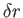per unit breadth (perpendicular to the figure), whereas that of the liquid with the solid is decreased byper unit breadth, and that of the liquid with the air is decreased by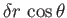per unit breadth. Thus, the net change in free energy per unit breadth is(3.17)

However, an equilibrium state is one which minimizes the free energy, implying that the previous expression is zero for arbitrary (small): that is,(3.18)

We conclude that, in equilibrium, the angle of contact,, between the liquid and the solid takes a fixed value that depends on the free energies per unit area at the liquid/air, liquid/solid, and air/solid interfaces. Note that the previous formula could also be obtained from the requirement that the various surface tension forces acting at the edge balance one another, assuming that it is really appropriate to interpretandas surface tensions when one of the media making up the interface is a solid.

As explained in Section 3.1, we would generally expectandto be positive. On the other hand,could be either positive or negative. Now, because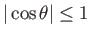, Equation (3.18) can only be solved whenlies in the range(3.19)

If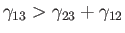then the angle of contact is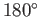, which corresponds to the case where the free energy at the liquid/solid interface is so large that the liquid does not wet the solid at all, but instead breaks up into beads on its surface. On the other hand, ifthen the angle of contact is, which corresponds to the case where the free energy at the liquid/solid interface is so small that the liquid completely wets the solid, spreading out indefinitely until it either covers the whole surface, or its thickness reaches molecular dimensions.

The angle of contact between water and glass typically lies in the rangeto, whereas that between mercury and glass is about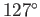(Batchelor 2000).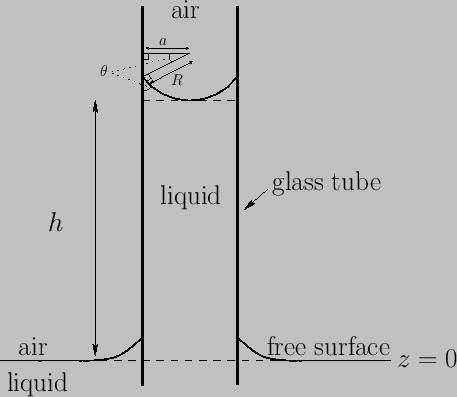Next: Jurin's Law Up: Surface Tension Previous: Capillary Length
Richard Fitzpatrick 2016-03-31